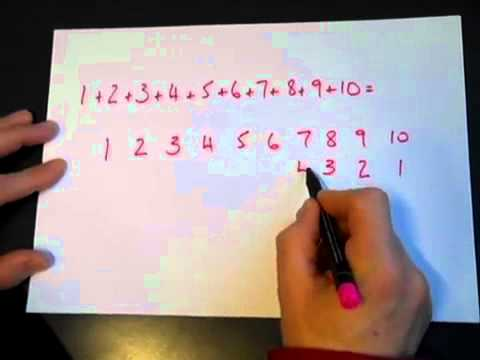### Submit a Resource

NRICH: Slick SummingWatch the video to see how Charlie works out the sum. Can you adapt his method?

In the video below, Charlie works out 1+2+3+4+5+6+7+8+9+10.

Can you see how his method works?

How could you adapt his method to work out the following sums?

1+2+3++19+20

1+2+3++99+100

40+41+42++99+100

Can Charlie’s method be adapted to sum sequences that don’t go up in ones?

1+3+5++17+19

2+4+6++18+20

42+44+46++98+100

Can you find an expression for the following sum?
1+2+3++(n1)+n

Notes and Background

If you enjoyed this problem you may be interested to read the article Clever Carl, which tells the story of the young Gauss working on sums like the ones in this problem.

Age 14 to 16

Algebra & Pre-Algebra, Patterning & Sequencing, Quadratic Equations
High School, Educator

## Organization

NRICH (University of Cambridge)

Challenge
Video